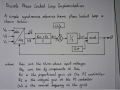# Phase Locked Loop Analysis: Where is this gain coming from?

Thread Starter

#### TechWise

Joined Aug 24, 2018
151
I'm following a tutorial on a simple phase locked loop design here:
I have also attached a diagram. When tuning the PI controller, it is necessary to determine the open loop transfer function. In the video, the abc-to-dq transform block is treated as a simple gain of Vg which is the magnitude of the input voltage. Can anyone explain why?

This is technically a control question but it's related to power electronics so mods should feel free to move.#### BobaMosfet

Joined Jul 1, 2009
1,776
@TechWise

"...Vg which is the magnitude of the input voltage...." <--think about that.

Thread Starter

#### TechWise

Joined Aug 24, 2018
151
@TechWise

"...Vg which is the magnitude of the input voltage...." <--think about that.
What's throwing me here is that the magnitude, Vg, is already accounted for as the signals entering from the left hand side are of the form Vg*sin(wt). This is why I'm unsure why you would multiply by the Vg a second time.

I think what might be happening is that we assume Vabc is constant, in which case varying theta would cause Vq to vary sinusoidally. However, if you assume that the change in theta is very very small then you could approximate the dq transform as linear and say that the dq transform of a small delta theta is equal to a small delta Vq.